Question

# Example Consider the following dice game. A pair of standard ( fair ) dice are repeatedly...

Example

Consider the following dice game. A pair of standard ( fair ) dice are repeatedly rolled. If a ’ 7 ’ comes up before an ’ 11 ’ , then the player wins, otherwise the player loses. Let W be the event that the player wins. Find P(W). To say the dice are fair is equivalent to assuming that Laplace’s rule holds and the 36 possible outcomes for a throw of the dice are equally likely. For convenience, an addition table is presented in Table 1. Table 1: Sum of Spots on Two Dice + 1 2 3 4 5 6 1 2 3 4 5 6 7 2 3 4 5 6 7 8 3 4 5 6 7 8 9 4 5 6 7 8 9 10 5 6 7 8 9 10 11 6 7 8 9 10 11 12

Solution

Let F be the event that the game ends on the ﬁrst roll; let L be the event that the game ends on some later roll. Write P(W) = P(W ∩F) + P(W ∩L) = P(W ∩F) + P(W|L)P(L) Now P(W∩F) is just the probability the ﬁrst roll is a seven, so P(W∩F) = 1 6. The game extends past the ﬁrst roll if the player neither wins nor loses on the ﬁrst roll, which is the probability the ﬁrst roll is neither a seven nor an eleven. So P(L) = 1−1 6− 1 18. Now here’s the part that requires a little thought: dice have no memory, hold no grudges, so the chances you eventually win aren’t any greater or smaller if the game goes to a second roll than they were when the player ﬁrst picked up the dice. Hence P(W|L) = P(W). Putting all this together, one has P(W) = 1 6 + P(W)(1− 1 6 − 1 18 ) 13 Solving for P(W) one obtains P(W) = 1 6 1 6 + 1 18 = 3 4

******please do the following exercise *******

Exercise 6.

. Generalize the last example. Let A and B be two disjoint events, e.g. rolling a seven and rolling an even number, respectively. If A < B is the event that A happens before B in repeated rolls,

1. show that P(A < B) = P(A) P(A) + P(B)

2. If 2A < B denotes the event at A happens twice before B happens, ﬁnd an expression for P(2A < B)

. 3. Find the probability that in repeated rolls of a pair of fair dice one rolls an eleven before two rolls of a seven occur.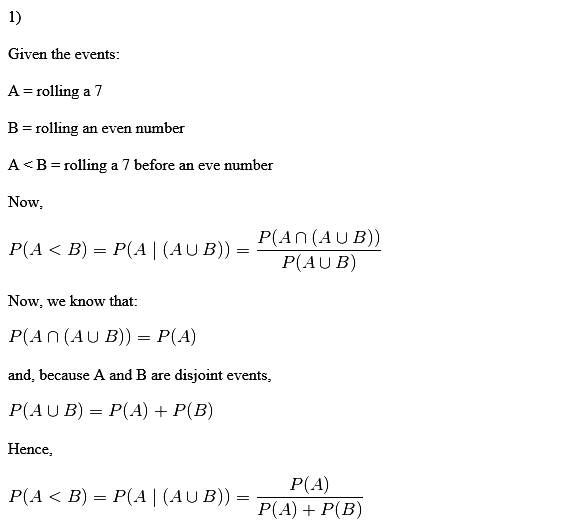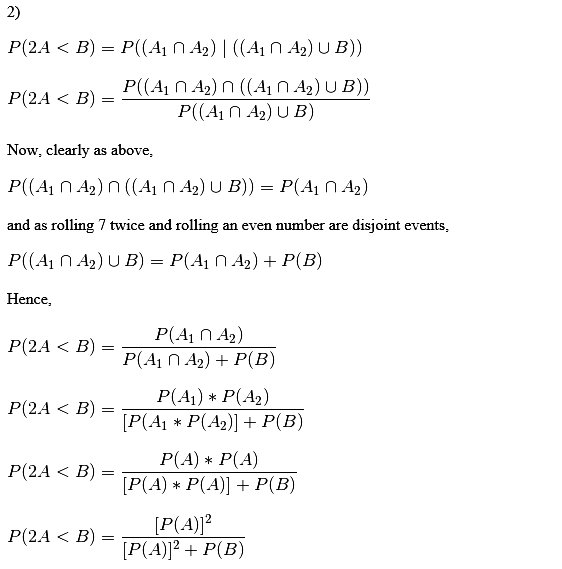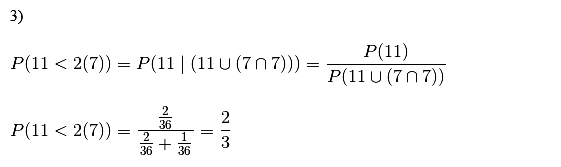#### Earn Coins

Coins can be redeemed for fabulous gifts.

Similar Homework Help Questions
• ### 2. "Craps" is a game played by rolling two fair dice. To play one round of...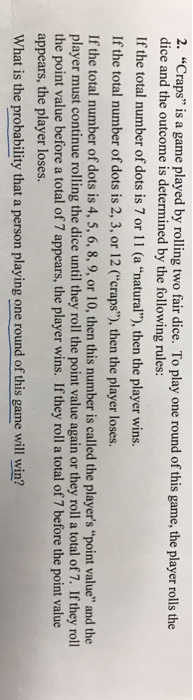2. "Craps" is a game played by rolling two fair dice. To play one round of this game, the player rolls the dice and the outcome is determined by the following rules: If the total number of dots is 7 or 11 (a "natural"), then the player wins. If the total number of dots is 2, 3, or 12 C'craps"), then the player loses. If the total number of dots is 4, 5, 6,8,9, or 10, then this number is...

• ### . In the carnival game Under-or-Over-Seven, a pair of fair dice is rolled once, and the...

. In the carnival game Under-or-Over-Seven, a pair of fair dice is rolled once, and the resulting sum determines whether the player wins or loses his or her bet. For example, the player can bet \$1 that the sum will be under 7—that is, 2, 3, 4, 5, or 6. For this bet, the player wins \$1 if the result is under 7 and loses \$1 if the outcome equals or is greater than 7. Similarly, the player can bet...

• ### 9. In the casino dice game Craps, players make wagers on a sequence of rolls of...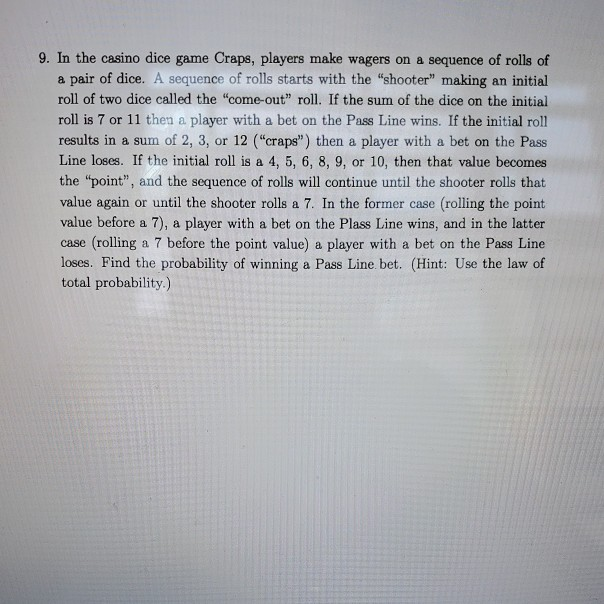9. In the casino dice game Craps, players make wagers on a sequence of rolls of a pair of dice. A sequence of rolls starts with the "shooter" making an initial roll of two dice called the "come-out” roll. If the sum of the dice on the initial roll is 7 or 11 then a player with a bet on the Pass Line wins. If the initial roll results in a sum of 2, 3, or 12 ("craps") then a...

• ### Bob plays a game in which he rolls a pair of fair 6-sided dice once. He...

Bob plays a game in which he rolls a pair of fair 6-sided dice once. He adds the number of dots on both dice to create a sum. He loses \$1 if he rolls a sum less than 5 doesn’t win or lose anything if he rolls a sum that is higher than 4, but less than 8 wins \$1 if he rolls a sum that’s higher than 7 but less than 12 wins \$2 if he rolls a sum...

• ### using python  Craps is a dice-based game played in many casinos. Like blackjack, a player...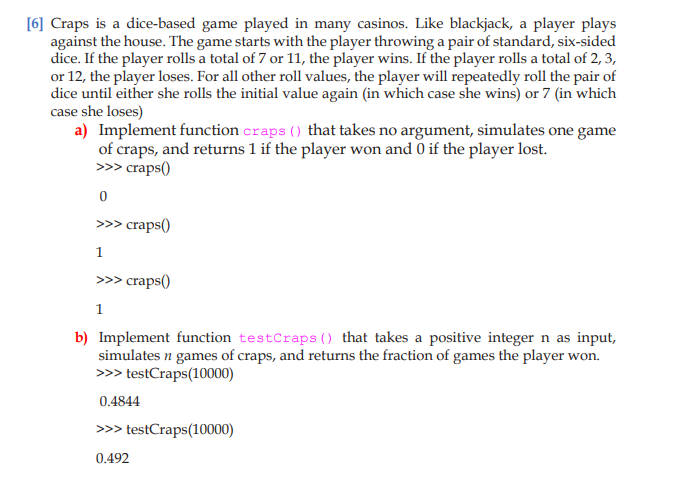using python  Craps is a dice-based game played in many casinos. Like blackjack, a player plays against the house. The game starts with the player throwing a pair of standard, six-sided dice. If the player rolls a total of 7 or 11, the player wins. If the player rolls a total of 2,3, or 12, the player loses. For all other roll values, the player will repeatedly roll the pair of dice until either she rolls the initial value...

• ### Bob and Doug are playing the following game. Bob starts by rolling two fair dice; if...

Bob and Doug are playing the following game. Bob starts by rolling two fair dice; if the sum of his dice is six, then he wins the game. If not, then Doug rolls the dice, and if the sum of his rolls is seven, then he wins the game. If neither player wins the game during the first round, then they repeat the process (with Bob going first) until someone wins a round. What is the probability that Bob wins...

• ### A dice game is played with two distinct 12 sided dice. It costs \$3 to roll...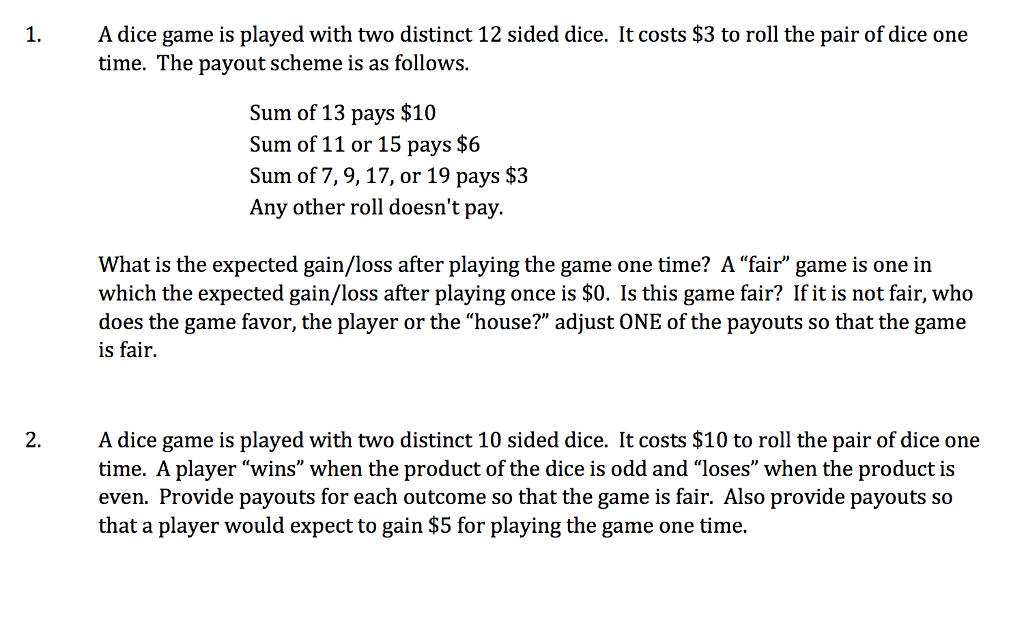A dice game is played with two distinct 12 sided dice. It costs \$3 to roll the pair of dice one time. The payout scheme is as follows 1. Sum of 13 pays \$10 Sum of 11 or 15 pays \$6 Sum of 7, 9, 17, or 19 pays \$3 Any other roll doesn't pay. What is the expected gain/loss after playing the game one time? A "fair" game is one in which the expected gain/loss after playing once is...

• ### Please i need helpe with this JAVA code. Write a Java program simulates the dice game...

Please i need helpe with this JAVA code. Write a Java program simulates the dice game called GAMECrap. For this project, assume that the game is being played between two players, and that the rules are as follows: Problem Statement One of the players goes first. That player announces the size of the bet, and rolls the dice. If the player rolls a 7 or 11, it is called a natural. The player who rolled the dice wins. 2, 3...

• ### In a dice game, the player independently rolls a fair red die and a fair green...

In a dice game, the player independently rolls a fair red die and a fair green die.The player wins if and only if the red die shows a 1, or 2, or 3, or if the total on the two dice is 11. What is the probability the player will win?

• ### 1 point) Three brothers play a game with a pair of fair (six-sided) dice. Scott will...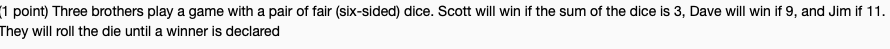1 point) Three brothers play a game with a pair of fair (six-sided) dice. Scott will win if the sum of the dice is 3, Dave will win if 9, and Jim if 11 They will roll the die until a winner is declared Part (c) Realizing this, theoretically, is a game that could go on forever..the three brothers decide that if no winner has been decided in three rolls or "turns" Scott will be deemed the winner. Let Y...